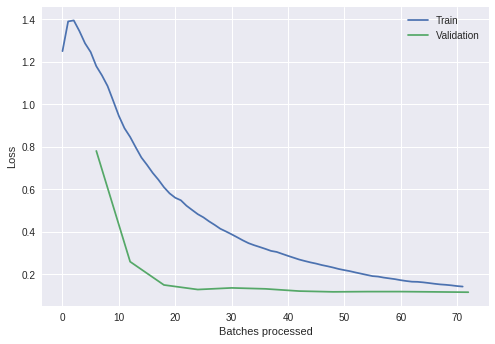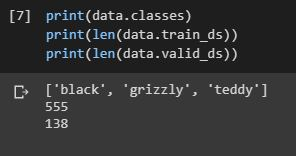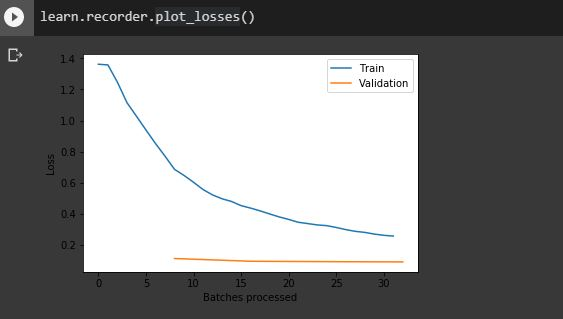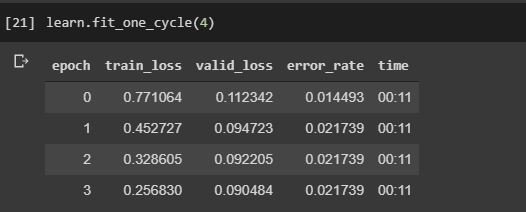# How to interpret train and validation losses vs epochs

Hi,

I am at Lesson 2.

When training a model, I oftentimes get train losses higher than validation losses. This is puzzling as I expect the validation loss to be higher in general. What is the reason of this behavior?

EDIT: In the lecture Jeremy mentions (49:00) that this situations happens when the number of epochs is too low or the learning rate too low.
In my case, I train a resnet34:

``````learn = create_cnn(data, models.resnet34, metrics=error_rate)
learn.fit_one_cycle(12, max_lr=3e-3)
``````

and the losses look like this:From the graph, we see that the validation error stops improving after 3-4 epochs. For the remaining epochs only the training error keeps decreasing. Is the model slightly overfitting here? Why the training losses are higher than the validation ones?

I also checked different learning rates. If I use higher ones (1e-2) the model does not converge to low error anymore. With lower learning rates, learning is slower, but still the validation losses are smaller than the training ones.

The dataset is fairly standard. It is firetrucks vs schoolbus vs ambulances downloaded from google images with more than 500 images in total (after clean-up):

``````>>> data.classes, data.c, len(data.train_ds), len(data.valid_ds)
(['ambulance', 'firetruck', 'schoolbus'], 3, 415, 103)
``````

If you want to try, the (cleaned) dataset is here:

Any suggestions to understand the cause of this behavior?

2 Likes

I found this thread discussing the same issue:

The problem is attributed to underfitting. But I am still unconvinced as underfitting means that the model has high bias, systematically gives non-correct predictions. Instead here the model gives good prediction on the validation set.

Hi,
I’m trying to classify between teddy, black and grizzly bears same as the lesson 2 notebook.I’m also faced with the same problem (i.e) my train loss is greater than the valid loss. As mentioned by jeremy this is due to underfitting (i.e) either you should increase the number of epochs or you should bump up the learning rate.
My results:I’m satisfied with the results as the error rate is close to 2% but what bugs me is that jeremy said when train loss is greater than valid loss it is due to underfitting. But how could it be underfitting as my performance on the validation set is good enough!!
Help me here!